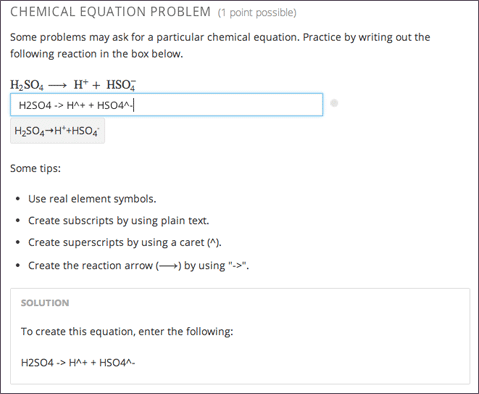# 10.6. Chemical Equation Problem¶

Note

EdX does not support this problem type.

The chemical equation problem type allows the learner to enter text that represents a chemical equation into a text box. The system converts that text into a chemical equation below the text box. The grader evaluates the learner’s response by using a Python script that you create and embed in the problem.Note

You can make a calculator available to your learners on every unit page. For more information, see Calculator Tool.

## 10.6.1. Create a Chemical Equation Problem¶

Chemical equation problems use MathJax to create formulas. For more information about using MathJax in Studio, see Using MathJax for Mathematics.

To create the above chemical equation problem, follow these steps.

1. In the unit where you want to create the problem, select Problem under Add New Component, and then select the Advanced tab.

3. In the component that appears, select Edit.

4. In the component editor, paste the code from below.

5. Select Save.

### 10.6.1.1. Sample Chemical Equation Problem Code¶

<problem>
<startouttext/>
<p>Some problems may ask for a particular chemical equation. Practice by writing out the following reaction in the box below.</p>

$$\text{H}_2\text{SO}_4 \longrightarrow \text { H}^+ + \text{ HSO}_4^-$$

<customresponse>
<chemicalequationinput size="50" label="Enter the chemical equation"/>

if chemcalc.chemical_equations_equal(submission, 'H2SO4 -> H^+ + HSO4^-'):
correct = ['correct']
else:
correct = ['incorrect']

</customresponse>
<p>Some tips:</p>
<ul>
<li>Use real element symbols.</li>
<li>Create subscripts by using plain text.</li>
<li>Create superscripts by using a caret (^).</li>
<li>Create the reaction arrow ($$\longrightarrow$$) by using "->".</li>
</ul>

<endouttext/>

<solution>
<div class="detailed-solution">
<p>Solution</p>
<p>To create this equation, enter the following:</p>
<p>H2SO4 -> H^+ + HSO4^-</p>
</div>
</solution>
</problem>


## 10.6.2. Chemical Equation Problem XML¶

### 10.6.2.1. Template¶

<problem>
<startouttext/>
<p>Problem text</p>

<customresponse>
<chemicalequationinput size="NUMBER" label="LABEL TEXT"/>

if chemcalc.chemical_equations_equal(submission, 'TEXT REPRESENTING CHEMICAL EQUATION'):
correct = ['correct']
else:
correct = ['incorrect']

</customresponse>

<endouttext/>

<solution>
<div class="detailed-solution">
<p>Solution or explanation text</p>
</div>
</solution>
</problem>


### 10.6.2.2. Tags¶

• <customresponse>: Indicates that this problem has a custom response.

• <chemicalequationinput>: Specifies that the answer to this problem is a chemical equation.

• <answer type=loncapa/python>: Contains the Python script that grades the problem.

Tag: <customresponse>

Indicates that this problem has a custom response. The <customresponse> tags must surround the <chemicalequation> tags.

Attributes

(none)

Children

• <chemicalequationinput>

• <answer>

Tag: <chemicalequationinput>

Indicates that the answer to this problem is a chemical equation and creates a response field where the learner enters an answer.

Attributes

 Attribute Description size Specifies the size of the response field, in characters. label (required) Contains the text of the principal question in the problem.

Children

(none)

Tag: <answer>

Contains the Python script that grades the problem.

Attributes

 Attribute Description type (required) Must be “loncapa/python”.

Children

(none)